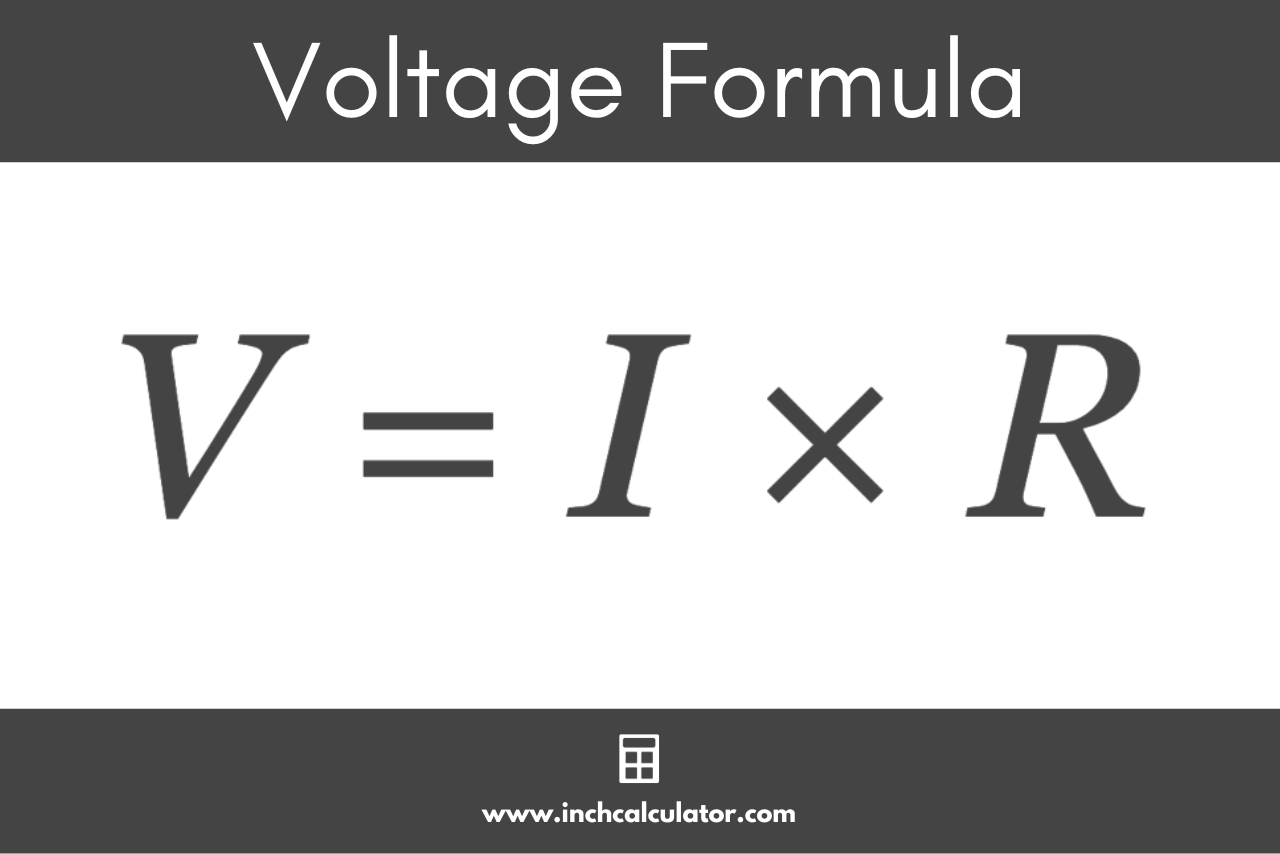# Voltage Calculator

Use the voltage calculator below to calculate the voltage given the current, power, or resistance.

## Calculation Formulas:

Learn how we calculated this below

## How to Calculate Voltage

Voltage is the potential difference between two points in an electrical circuit, measured in volts. It is the measure of the electric potential to move an electric charge in a circuit.

You can calculate the voltage in a circuit using Ohm’s Law, which states that the current through a circuit element is directly proportional to the potential difference applied to it and inversely proportional to the resistance.

### Voltage Formula

The Ohm’s Law formula states I = V/R, where I is the current through the circuit (in amps), V is the potential difference across the circuit (in volts), and R is the resistance of the circuit (in ohms).

By rearranging this formula, you can calculate the voltage by multiplying the current flowing through a circuit by the resistance of the circuit. The formula to calculate voltage is:

V = I × R

Thus, the voltage across the circuit V is equal to the circuit flowing through the circuit I times the resistance of the circuit R.This is the formula that is used to convert amps to volts.

## How to Calculate the Voltage Using Watts

You can also calculate the voltage in a circuit using Watt’s Law power formula. If you know the power in watts drawn through a circuit and the current, the formula to calculate the voltage is:

V = P / I

The voltage of the circuit V is equal to the power drawn through the circuit P divided by the current flowing through the circuit I.

This is the formula that is used to convert watts to volts.

## References

1. Brandon Mitchell, Robert Ekey, Roy McCullough, and William Reitz, A Fan-tastic Quantitative Exploration of Ohm’s Law, https://aapt.scitation.org/doi/full/10.1119/1.5021431
2. National Institute of Standards and Technology, Ampere: The Present, https://www.nist.gov/si-redefinition/ampere-present